# Dark Energy and Accelerated Expansion of the Universe

01/01/198008/10/1998View on timeline

Dark energy is the repulsive force that is the dominant component (69.4 percent) of the universe. The remaining portion of the universe consists of ordinary matter and dark matter. Dark energy, in contrast to both forms of matter, is relatively uniform in time and space and is gravitationally repulsive, not attractive, within the volume it occupies. The nature of dark energy is still not well understood.

Dark energy is an unknown form of energy which is hypothesized to permeate all of space, tending to accelerate the expansion of the universe. Dark energy is the most accepted hypothesis to explain the observations since the 1990s indicating that the universe is expanding at an accelerating rate.

Assuming that the standard model of cosmology is correct, the best current measurements indicate that dark energy contributes 68% of the total energy in the present-day observable universe. The mass–energy of dark matter and ordinary (baryonic) matter contribute 27% and 5%, respectively, and other components such as neutrinos and photons contribute a very small amount. The density of dark energy (~ 7×10−30 g/cm3) is very low, much less than the density of ordinary matter or dark matter within galaxies. However, it dominates the mass–energy of the universe because it is uniform across space.

Two proposed forms for dark energy are the cosmological constant, representing a constant energy density filling space homogeneously, and scalar fieldssuch as quintessence or moduli, dynamic quantities whose energy density can vary in time and space. Contributions from scalar fields that are constant in space are usually also included in the cosmological constant. The cosmological constant can be formulated to be equivalent to the zero-point radiation of space i.e. the vacuum energy. Scalar fields that change in space can be difficult to distinguish from a cosmological constant because the change may be extremely slow.

#### History of discovery and previous speculation

Einstein's cosmological constant

The "cosmological constant" is a constant term that can be added to Einstein's field equation of general relativity. If considered as a "source term" in the field equation, it can be viewed as equivalent to the mass of empty space (which conceptually could be either positive or negative), or "vacuum energy".

The cosmological constant was first proposed by Einstein as a mechanism to obtain a solution of the gravitational field equation that would lead to a static universe, effectively using dark energy to balance gravity. Einstein gave the cosmological constant the symbol Λ (capital lambda).

The mechanism was an example of fine-tuning, and it was later realized that Einstein's static universe would not be stable: local inhomogeneities would ultimately lead to either the runaway expansion or contraction of the universe. The equilibrium is unstable: if the universe expands slightly, then the expansion releases vacuum energy, which causes yet more expansion. Likewise, a universe which contracts slightly will continue contracting. These sorts of disturbances are inevitable, due to the uneven distribution of matter throughout the universe. Further, observations made by Edwin Hubble in 1929 showed that the universe appears to be expanding and not static at all. Einstein reportedly referred to his failure to predict the idea of a dynamic universe, in contrast to a static universe, as his greatest blunder.

Inflationary dark energy

Alan Guth and Alexei Starobinsky proposed in 1980 that a negative pressure field, similar in concept to dark energy, could drive cosmic inflation in the very early universe. Inflation postulates that some repulsive force, qualitatively similar to dark energy, resulted in an enormous and exponential expansion of the universe slightly after the Big Bang. Such expansion is an essential feature of most current models of the Big Bang. However, inflation must have occurred at a much higher energy density than the dark energy we observe today and is thought to have completely ended when the universe was just a fraction of a second old. It is unclear what relation, if any, exists between dark energy and inflation. Even after inflationary models became accepted, the cosmological constant was thought to be irrelevant to the current universe.

Nearly all inflation models predict that the total (matter+energy) density of the universe should be very close to the critical density. During the 1980s, most cosmological research focused on models with critical density in matter only, usually 95% cold dark matter and 5% ordinary matter (baryons). These models were found to be successful at forming realistic galaxies and clusters, but some problems appeared in the late 1980s: in particular, the model required a value for the Hubble constant lower than preferred by observations, and the model under-predicted observations of large-scale galaxy clustering. These difficulties became stronger after the discovery of anisotropy in the cosmic microwave background by the COBE spacecraft in 1992, and several modified CDM models came under active study through the mid-1990s: these included the Lambda-CDM model and a mixed cold/hot dark matter model. The first direct evidence for dark energy came from supernova observations in 1998 of accelerated expansion in Riess et al. and in Perlmutter et al., and the Lambda-CDM model then became the leading model. Soon after, dark energy was supported by independent observations: in 2000, the BOOMERanG and Maxima cosmic microwave background experiments observed the first acoustic peak in the CMB, showing that the total (matter+energy) density is close to 100% of critical density. Then in 2001, the 2dF Galaxy Redshift Survey gave strong evidence that the matter density is around 30% of critical. The large difference between these two supports a smooth component of dark energy making up the difference. Much more precise measurements from WMAP in 2003–2010 have continued to support the standard model and give more accurate measurements of the key parameters.

The term "dark energy", echoing Fritz Zwicky's "dark matter" from the 1930s, was coined by Michael Turner in 1998.

Change in expansion over time

High-precision measurements of the expansion of the universe are required to understand how the expansion rate changes over time and space. In general relativity, the evolution of the expansion rate is estimated from the curvature of the universe and the cosmological equation of state (the relationship between temperature, pressure, and combined matter, energy, and vacuum energy density for any region of space). Measuring the equation of state for dark energy is one of the biggest efforts in observational cosmology today. Adding the cosmological constant to cosmology's standard FLRW metric leads to the Lambda-CDM model, which has been referred to as the "standard model of cosmology" because of its precise agreement with observations.

As of 2013, the Lambda-CDM model is consistent with a series of increasingly rigorous cosmological observations, including the Planck spacecraft and the Supernova Legacy Survey. First results from the SNLS reveal that the average behavior (i.e., equation of state) of dark energy behaves like Einstein's cosmological constant to a precision of 10%. Recent results from the Hubble Space Telescope Higher-Z Team indicate that dark energy has been present for at least 9 billion years and during the period preceding cosmic acceleration.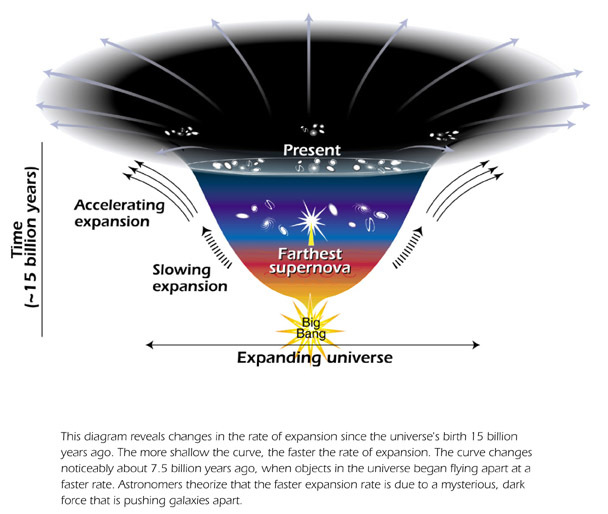Diagram representing the accelerated expansion of the universe due to dark energy.

#### Evidence of existence

The evidence for dark energy is indirect but comes from three independent sources:

• Distance measurements and their relation to redshift, which suggest the universe has expanded more in the last half of its life.
• The theoretical need for a type of additional energy that is not matter or dark matter to form the observationally flat universe (absence of any detectable global curvature).
• Measures of large-scale wave-patterns of mass density in the universe.

Supernovae

In 1998, the High-Z Supernova Search Team published observations of Type Ia ("one-A") supernovae. In 1999, the Supernova Cosmology Project followed by suggesting that the expansion of the universe is accelerating. The 2011 Nobel Prize in Physics was awarded to Saul Perlmutter, Brian P. Schmidt, and Adam G. Riess for their leadership in the discovery.

Since then, these observations have been corroborated by several independent sources. Measurements of the cosmic microwave background, gravitational lensing, and the large-scale structure of the cosmos, as well as improved measurements of supernovae, have been consistent with the Lambda-CDM model. Some people argue that the only indications for the existence of dark energy are observations of distance measurements and their associated redshifts. Cosmic microwave background anisotropies and baryon acoustic oscillations serve only to demonstrate that distances to a given redshift are larger than would be expected from a "dusty" Friedmann–Lemaître universe and the local measured Hubble constant.

Supernovae are useful for cosmology because they are excellent standard candles across cosmological distances. They allow researchers to measure the expansion history of the universe by looking at the relationship between the distance to an object and its redshift, which gives how fast it is receding from us. The relationship is roughly linear, according to Hubble's law. It is relatively easy to measure redshift, but finding the distance to an object is more difficult. Usually, astronomers use standard candles: objects for which the intrinsic brightness, or absolute magnitude, is known. This allows the object's distance to be measured from its actual observed brightness, or apparent magnitude. Type Ia supernovae are the best-known standard candles across cosmological distances because of their extreme and consistent luminosity.

Recent observations of supernovae are consistent with a universe made up 71.3% of dark energy and 27.4% of a combination of dark matter and baryonic matter.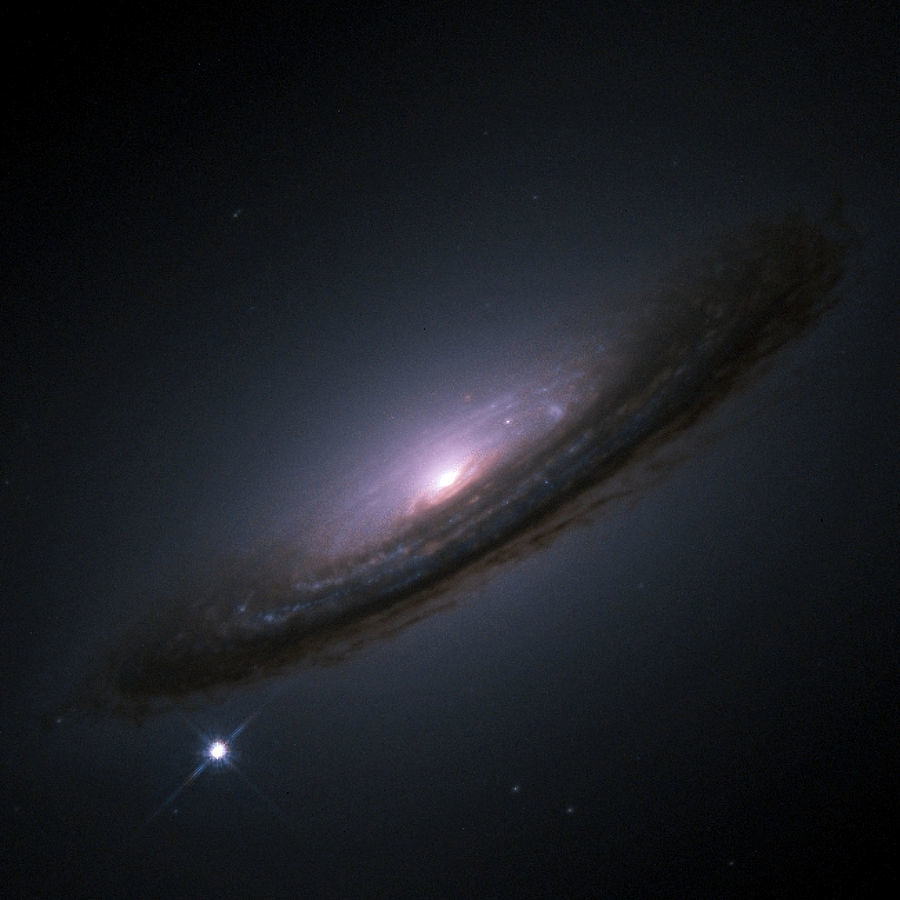A Type Ia supernova (bright spot on the bottom-left) near a galaxy.

Cosmic microwave background

The existence of dark energy, in whatever form, is needed to reconcile the measured geometry of space with the total amount of matter in the universe. Measurements of cosmic microwave background (CMB) anisotropies indicate that the universe is close to flat. For the shape of the universe to be flat, the mass-energy density of the universe must be equal to the critical density. The total amount of matter in the universe (including baryons and dark matter), as measured from the CMB spectrum, accounts for only about 30% of the critical density. This implies the existence of an additional form of energy to account for the remaining 70%. The Wilkinson Microwave Anisotropy Probe (WMAP) spacecraft seven-year analysis estimated a universe made up of 72.8% dark energy, 22.7% dark matter, and 4.5% ordinary matter. Work done in 2013 based on the Planck spacecraft observations of the CMB gave a more accurate estimate of 68.3% dark energy, 26.8% dark matter, and 4.9% ordinary matter.Estimated division of total energy in the universe into matter, dark matter and dark energy based on...

#### Theories of dark energy

Dark energy's status as a hypothetical force with unknown properties makes it a very active target of research. The problem is attacked from a great variety of angles, such as modifying the prevailing theory of gravity (general relativity), attempting to pin down the properties of dark energy, and finding alternative ways to explain the observational data.

Cosmological constant

The simplest explanation for dark energy is that it is an intrinsic, fundamental energy of space. This is the cosmological constant, usually represented by the Greek letter Λ (Lambda, hence Lambda-CDM model). Since energy and mass are related according to the equation E = mc2, Einstein's theory of general relativity predicts that this energy will have a gravitational effect. It is sometimes called a vacuum energy because it is the energy density of empty vacuum.

The cosmological constant has negative pressure equal to its energy density and so causes the expansion of the universe to accelerate. The reason a cosmological constant has negative pressure can be seen from classical thermodynamics. In general, energy must be lost from inside a container (the container must do work on its environment) in order for the volume to increase. Specifically, a change in volume dV requires work done equal to a change of energy −P dV, where P is the pressure. But the amount of energy in a container full of vacuum actually increases when the volume increases, because the energy is equal to ρV, where ρ is the energy density of the cosmological constant. Therefore, P is negative and, in fact, P = −ρ.

There are two major advantages for the cosmological constant. The first is that it is simple. Einstein had in fact introduced this term in his original formulation of general relativity such as to get a static universe. Although he later discarded the term after Hubble found that the universe is expanding, a nonzero cosmological constant can act as dark energy, without otherwise changing the Einstein field equations. The other advantage is that there is a natural explanation for its origin. Most quantum field theories predict vacuum fluctuations that would give the vacuum this sort of energy. This is related to the Casimir effect, in which there is a small suction into regions where virtual particles are geometrically inhibited from forming (e.g. between plates with tiny separation).

A major outstanding problem is that the same quantum field theories predict a huge cosmological constant, more than 100 orders of magnitude too large. This would need to be almost, but not exactly, cancelled by an equally large term of the opposite sign. Some supersymmetric theories require a cosmological constant that is exactly zero, which does not help because supersymmetry must be broken.

Nonetheless, the cosmological constant is the most economical solution to the problem of cosmic acceleration. Thus, the current standard model of cosmology, the Lambda-CDM model, includes the cosmological constant as an essential feature.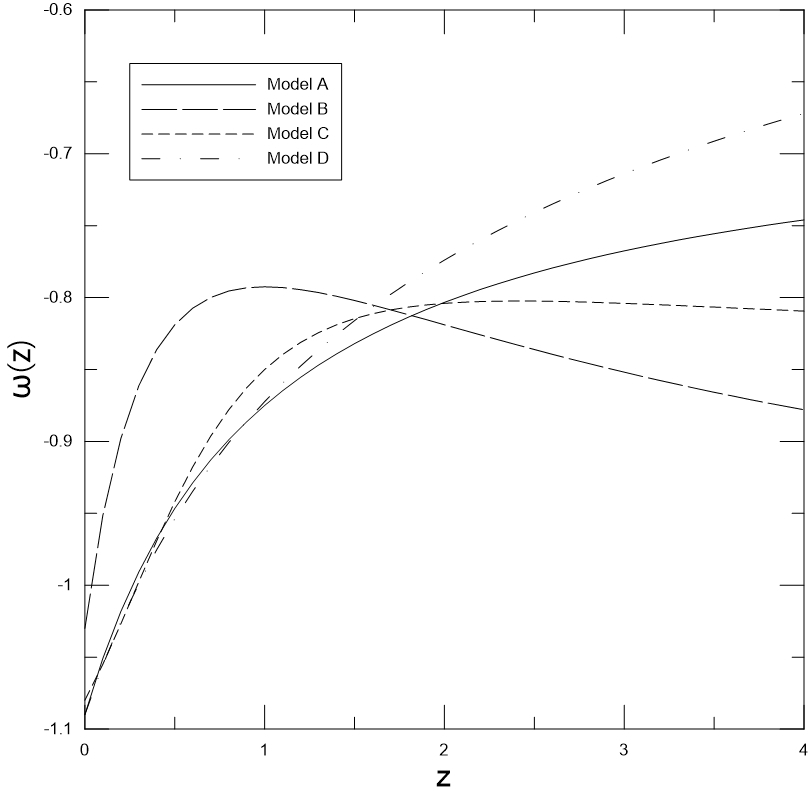The equation of state of Dark Energy for 4 common models by Redshift. A: CPL Model, B: Jassal Model,...

Quintessence

In quintessence models of dark energy, the observed acceleration of the scale factor is caused by the potential energy of a dynamical field, referred to as quintessence field. Quintessence differs from the cosmological constant in that it can vary in space and time. In order for it not to clump and form structure like matter, the field must be very light so that it has a large Compton wavelength.

No evidence of quintessence is yet available, but it has not been ruled out either. It generally predicts a slightly slower acceleration of the expansion of the universe than the cosmological constant. Some scientists think that the best evidence for quintessence would come from violations of Einstein's equivalence principle and variation of the fundamental constants in space or time. Scalar fields are predicted by the Standard Model of particle physics and string theory, but an analogous problem to the cosmological constant problem (or the problem of constructing models of cosmological inflation) occurs: renormalization theory predicts that scalar fields should acquire large masses.

The coincidence problem asks why the acceleration of the Universe began when it did. If acceleration began earlier in the universe, structures such as galaxies would never have had time to form, and life, at least as we know it, would never have had a chance to exist. Proponents of the anthropic principle view this as support for their arguments. However, many models of quintessence have a so-called "tracker" behavior, which solves this problem. In these models, the quintessence field has a density which closely tracks (but is less than) the radiation density until matter-radiation equality, which triggers quintessence to start behaving as dark energy, eventually dominating the universe. This naturally sets the low energy scale of the dark energy.

In 2004, when scientists fit the evolution of dark energy with the cosmological data, they found that the equation of state had possibly crossed the cosmological constant boundary (w = −1) from above to below. A No-Go theorem has been proved that gives this scenario at least two degrees of freedom as required for dark energy models. This scenario is so-called Quintom scenario.

Some special cases of quintessence are phantom energy, in which the energy density of quintessence actually increases with time, and k-essence (short for kinetic quintessence) which has a non-standard form of kinetic energy such as a negative kinetic energy. They can have unusual properties: phantom energy, for example, can cause a Big Rip.

Interacting dark energy

This class of theories attempts to come up with an all-encompassing theory of both dark matter and dark energy as a single phenomenon that modifies the laws of gravity at various scales. This could, for example, treat dark energy and dark matter as different facets of the same unknown substance, or postulate that cold dark matter decays into dark energy. Another class of theories that unifies dark matter and dark energy are suggested to be covariant theories of modified gravities. These theories alter the dynamics of the space-time such that the modified dynamic stems what have been assigned to the presence of dark energy and dark matter.

Variable dark energy models

The density of dark energy might have varied in time over the history of the universe. Modern observational data allow for estimates of the present density. Using baryon acoustic oscillations, it is possible to investigate the effect of dark energy in the history of the Universe, and constrain parameters of the equation of state of dark energy. To that end, several models have been proposed. One of the most popular models is the Chevallier–Polarski–Linder model (CPL). Some other common models are, (Barboza & Alcaniz. 2008), (Jassal et al. 2005), (Wetterich. 2004), (Oztas et al. 2018).

Observational skepticism

Some alternatives to dark energy aim to explain the observational data by a more refined use of established theories. In this scenario, dark energy doesn't actually exist, and is merely a measurement artifact. For example, if we are located in an emptier-than-average region of space, the observed cosmic expansion rate could be mistaken for a variation in time, or acceleration. A different approach uses a cosmological extension of the equivalence principle to show how space might appear to be expanding more rapidly in the voids surrounding our local cluster. While weak, such effects considered cumulatively over billions of years could become significant, creating the illusion of cosmic acceleration, and making it appear as if we live in a Hubble bubble. Yet other possibilities are that the accelerated expansion of the universe is an illusion caused by the relative motion of us to the rest of the universe, or that the supernovae sample size used wasn't large enough.

REFERENCES

Physics Today. Available in: https://physicstoday.scitation.org/do/10.1063/PT.6.6.20180108a/full/. Access in: 12/12/2018.

Space. Available in: https://www.space.com/6230-mysterious-dark-energy-confirmed-method.html. Access in: 12/12/2018.

Encyclopædia Britannica. Available in: https://www.britannica.com/science/dark-energy. Access in: 12/12/2018.

Wikipedia. Available in: https://en.wikipedia.org/wiki/Dark_energy. Access in: 12/12/2018.

Comment

Author

Info

Published in 13/12/2018

Updated in 19/02/2021

All events in the topic Astronomy and Cosmology:

Invalid DateThe Great Debate
Invalid DateLemaître theory of the expansion of the Universe
Invalid DateExpansion of the universe
Invalid Date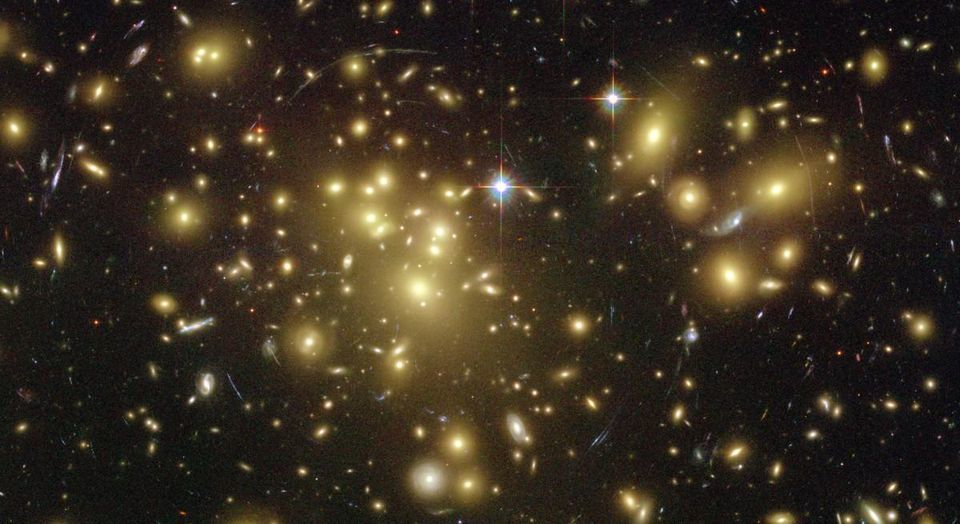Dark Matter
Invalid Date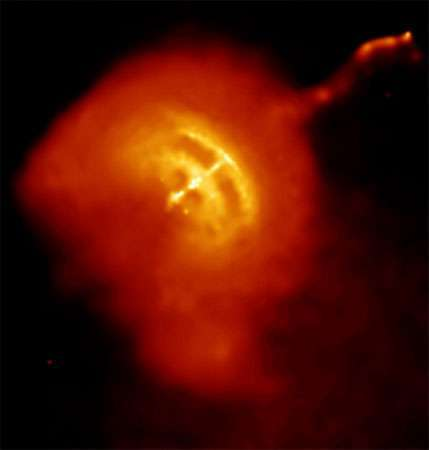Prediction of Neutron Stars
Invalid Date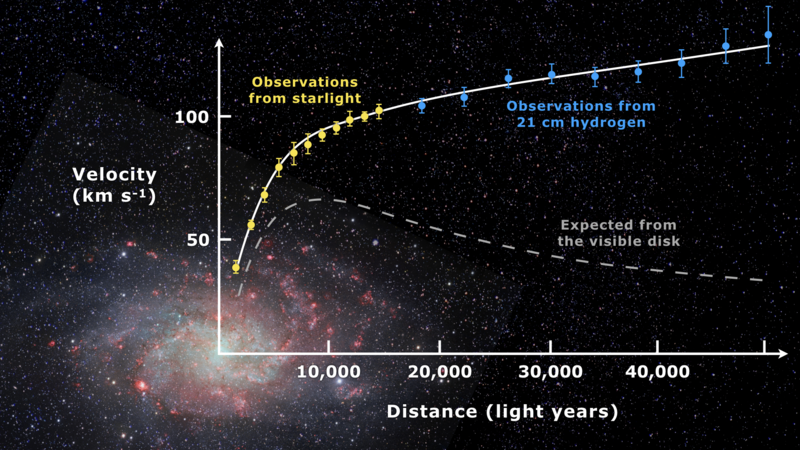Galaxy rotation curve
Invalid Date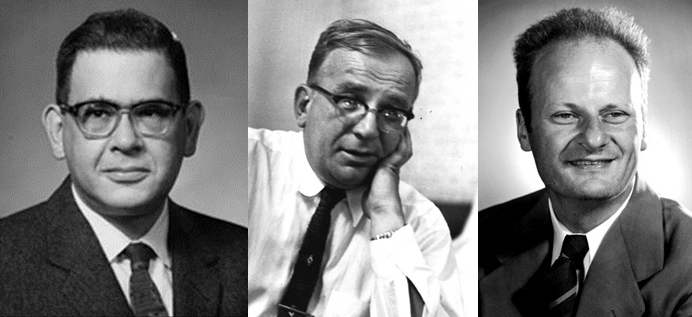Primordial Nucleosynthesis
Invalid DateDiscovery of cosmic background radiation
Invalid DateDiscovery of Neutrons Stars
Invalid DateDark Energy and Accelerated Expansion of the Universe
Invalid Date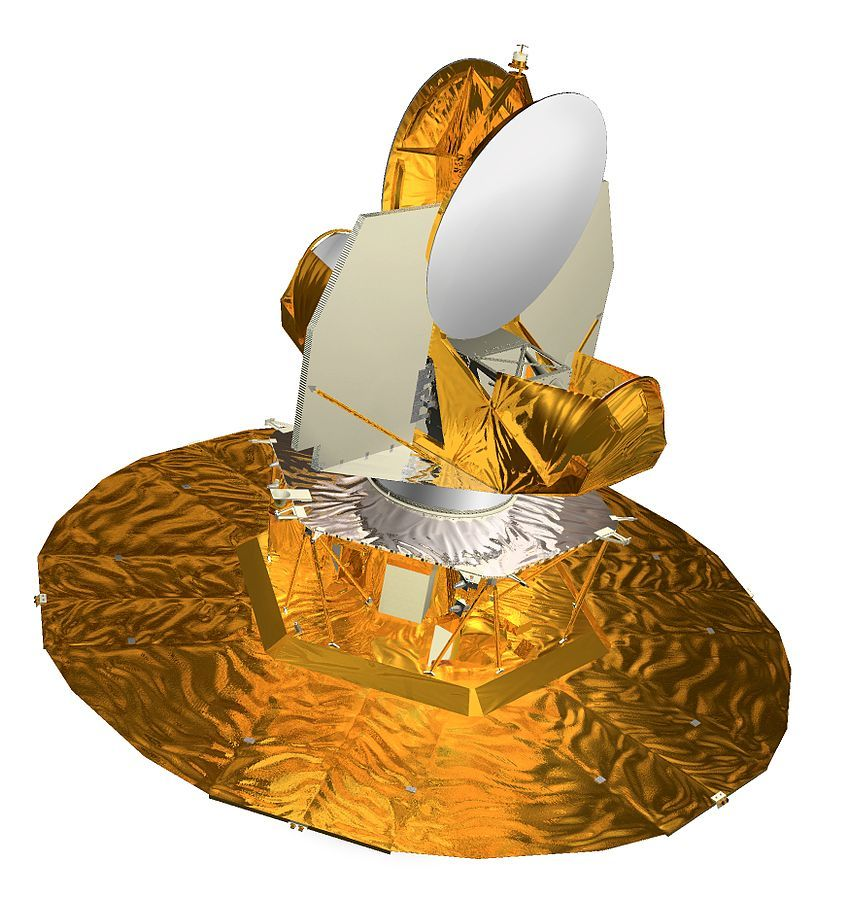WMAP Satellite
Invalid DatePlanck Satellite
Invalid DateBaryon acoustic oscillations
Invalid Date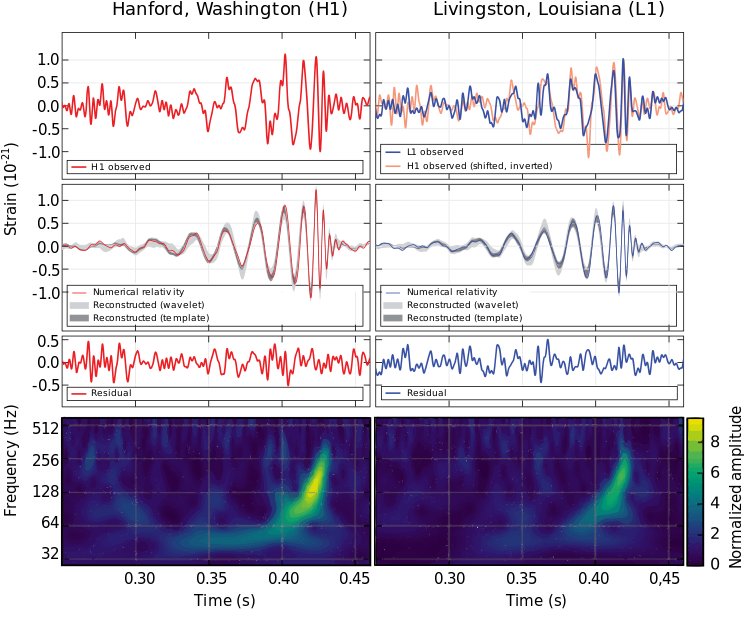Gravitational waves of two black holes
Invalid DateGravitational waves of two neutron stars